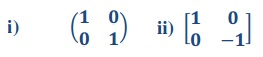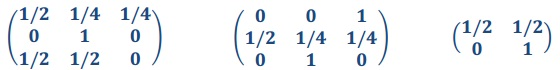Markov process and irreducible matrix

Question 1:

a) Describe about Markov process, ergodic chain and irreducible matrix?

b) which of the given matrices are stochastic?Question 2:

a) state and prove changing stakes result x2

b) If p = 1/3, q = 1/2, z =1, a = 1000 prove that dz = 999.

Question 3:

a) A fair coin is tossed repeatedly. If Xn denote the maximum number of numbers occurring in the first n tosses, find the transition probability matrx P of the Markov chain. Also find P2 and P (X2 = 6)

b) which of the given matrices are regular.Question 4: Three boys A, B and C are throwing a ball to each other. A always throws the ball to B and B always throws to C; but C is just as likely to throw the ball to B as to A. Show that the process is Markovian. Find out the transition matrix and categorize the states.

#### Solution Preview :

##### Reference No:- TGS03278

Now Priced at \$45 (50% Discount)

Recommended (96%)

Rated (4.8/5)